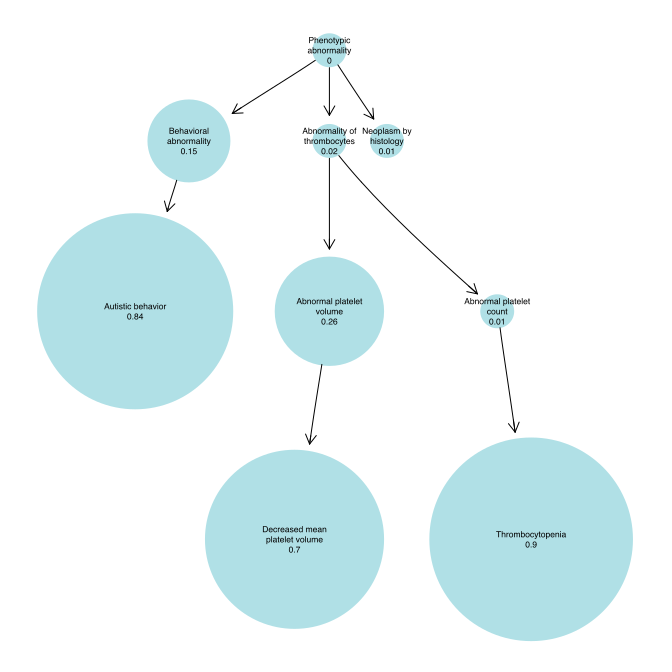# Similarity Regression - Introduction

#### 2016-10-15

SimReg has a function sim_reg for performing Bayesian Similarity Regression . It returns an estimated probability of an association between ontological term sets and a binary variable, and conditional on the association: a characteristic ontological profile, such that ontological similarity to the profile increases the probability of the binary variable taking the value TRUE. The procedure has been used in the context of linking an ontologically encoded phenotype (as HPO terms) to a binary genotype (indicating the presence or absence of a rare variant within given genes) , so in this guide, we’ll adopt the same theme.

The function accepts arguments including logical response variable y, ontologically encoded predictor variable x, and additional arguments for tuning the compromise between execution speed and precision for the procedure.

It returns an object of class sim_reg_output, which contains the pertinent information about the results of the inference. Of particular interest is the estimated probability of association, i.e. the probability that model selection indicator gamma = 1. The function prob_association can be used on the output to obtain such and estimate. Also, the posterior distribution of the characteristic ontological profile phi may be of interest, for which the function get_term_marginals can be used.

To set up an environment where we can run a simple example, we need an ontology_index object. The ontologyIndex package contains such an object - the Human Phenotype Ontology, hpo - so we load the package and the data, and proceed to create an HPO profile template and an enclosing set of terms, terms, from which we’ll generate random term sets upon which to apply the function. In our setting, we’ll interpret this HPO profile template as the typical phenotype of a hypothetical disease. We set template to the set HP:0005537, HP:0000729 and HP:0001873, corresponding to phenotype abnormalities ‘Decreased mean platelet volume’, ‘Autistic behavior’ and ‘Thrombocytopenia’ respectively.

library(ontologyIndex)
library(SimReg)
data(hpo)
set.seed(1)

template <- c("HP:0005537", "HP:0000729", "HP:0001873")
terms <- get_ancestors(hpo, c(template, sample(hpo\$id, size=50)))

First, we’ll do an example where there is no association between x and y, and then one where there is an association.

In the example with no association, we’ll fix y, with 10 TRUEs and generate the x randomly, with each set of ontological terms determined by sampling 5 random terms from terms.

y <- c(rep(TRUE, 10), rep(FALSE, 90))
x <- replicate(simplify=FALSE, n=100, expr=minimal_set(hpo, sample(terms, size=5)))

Thus, our input data looks like:

y
##     TRUE  TRUE  TRUE  TRUE  TRUE  TRUE  TRUE  TRUE  TRUE  TRUE FALSE
##   FALSE FALSE FALSE FALSE FALSE FALSE FALSE FALSE FALSE FALSE FALSE
##   FALSE FALSE FALSE FALSE FALSE FALSE FALSE FALSE FALSE FALSE FALSE
##   FALSE FALSE FALSE FALSE FALSE FALSE FALSE FALSE FALSE FALSE FALSE
##   FALSE FALSE FALSE FALSE FALSE FALSE FALSE FALSE FALSE FALSE FALSE
##   FALSE FALSE FALSE FALSE FALSE FALSE FALSE FALSE FALSE FALSE FALSE
##   FALSE FALSE FALSE FALSE FALSE FALSE FALSE FALSE FALSE FALSE FALSE
##   FALSE FALSE FALSE FALSE FALSE FALSE FALSE FALSE FALSE FALSE FALSE
##   FALSE FALSE FALSE FALSE FALSE FALSE FALSE FALSE FALSE FALSE FALSE
##  FALSE
head(x)
## []
##  "HP:0006660" "HP:0030157" "HP:0001697" "HP:0011792" "HP:0002814"
##
## []
##  "HP:0001844" "HP:0003296" "HP:0010760" "HP:0001155" "HP:0000245"
##
## []
##  "HP:0010244" "HP:0011277" "HP:0045037" "HP:0012372" "HP:0003468"
##
## []
##  "HP:0002011" "HP:0006660" "HP:0004313" "HP:0040068" "HP:0012612"
##
## []
##  "HP:0011912" "HP:0011492" "HP:0006711"
##
## []
##  "HP:0010577" "HP:0012612" "HP:0030791" "HP:0001977" "HP:0000309"

Now we can call the sim_reg function to estimate the probability of an association (note: by default, the probability of an association has a prior of 0.05 and this can be set by passing a gamma_prior_prob argument), and print the mean posterior value of gamma, corresponding to our estimated probability of association.

no_assoc <- sim_reg(ontology=hpo, x=x, y=y)
prob_association(no_assoc)
##  0.0005632741

We note that there is a low probability of association. Now, we sample x conditional on y, so that if y[i] == TRUE, then x has 2 out of the 3 terms in template added to its profile.

x_assoc <- lapply(y, function(y_i) minimal_set(hpo, c(
sample(terms, size=5), if (y_i) sample(template, size=2))))

If we look again at the first few values in x for which y[i] == TRUE, we notice that they contain terms from the template.

head(x_assoc)
## []
##  "HP:0009811" "HP:0009488" "HP:0010701" "HP:0000729" "HP:0005537"
##
## []
##  "HP:0002086" "HP:0012649" "HP:0030777" "HP:0010095" "HP:0000729"
##  "HP:0001873"
##
## []
##  "HP:0009543" "HP:0012881" "HP:0006702" "HP:0010795" "HP:0001873"
##  "HP:0005537"
##
## []
##  "HP:0009120" "HP:0030063" "HP:0012647" "HP:0010343" "HP:0001873"
##  "HP:0005537"
##
## []
##  "HP:0002977" "HP:0000292" "HP:0000759" "HP:0011338" "HP:0000925"
##  "HP:0005537" "HP:0000729"
##
## []
##  "HP:0009120" "HP:0010342" "HP:0004426" "HP:0010319" "HP:0000729"
##  "HP:0005537"

Now we run the procedure again with the new x and y and print the mean posterior value of gamma.

assoc <- sim_reg(ontology=hpo, x=x_assoc, y=y)
prob_association(assoc)
##  0.9999682

We note that we infer a higher probability of association. We can also visualise the estimated characteristic ontological profile, using the function plot_term_marginals, and note that the inferred characteristic phenotype corresponds well to template.

plot_term_marginals(hpo, get_term_marginals(assoc), max_terms=8, fontsize=30)